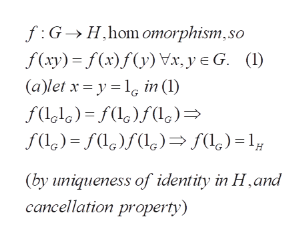# 1*. Let f GH be a group homomorphism. Prove:(a) If l is the identity of G then /(lg) is the identity of H(b) If x E G then f(x1) = f(x)-1

Question
1 viewshelp_outlineImage Transcriptionclose1*. Let f G H be a group homomorphism. Prove: (a) If l is the identity of G then /(lg) is the identity of H (b) If x E G then f(x1) = f(x)-1 fullscreen
check_circle

Step 1

To prove the required properties of a group homomorphism

Step 2help_outlineImage TranscriptionclosefGH,hom omorphism,so f(xy) f(x)(yVx, y G. () (a)let x yl in (l) fl)f()) (by uniqueness of identity in Hand cancellation property) fullscreen

### Want to see the full answer?

See Solution

#### Want to see this answer and more?

Solutions are written by subject experts who are available 24/7. Questions are typically answered within 1 hour.*

See Solution
*Response times may vary by subject and question.
Tagged in

### Math